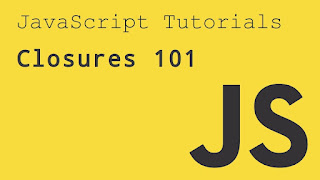# JavaScript closuresA closure can be defined as a JavaScript feature in which the inner function has access to the outer function variable. In JavaScript, every time a closure is created with the creation of a function.

The closure has three scope chains listed as follows:

• Access to the variables of the outer function.

Let's understand the closure by using an example.

### Example1

Code
1. <!DOCTYPE html>
2. <html>
4. <script>
5. function fun()
6. {
7. var a = 4; // 'a' is the local variable, created by the fun()
8. function innerfun() // the innerfun() is the inner function, or a closure
9. {
10. return a;
11. }
12. return innerfun;
13. }
14. var output = fun();
15. document.write(output());
16. document.write(" ");
17. document.write(output());
18. </script>
20. <body>
21.
22. </body>
23. </html>

In the above program we have two functions: fun() and innerfun(). The function fun() creates the local variable a and the function innerfun(). The inner function innerfun() is only present in the body of fun(). The inner function can access the outer function's variable, so the function innerfun() can access the variable 'a', which is declared and defined in fun().

This is the closure in action in which the inner function can have access to the global variables and outer function variables.

The entire body of function innerfun() is returned and stored in the variable output, due to the statement return innerfun. The inner function is not executed only by using the return statement; it is executed only when followed by the braces ().

In the output, the code will display the value of the variable 'a', defined in the parent function.

Now, there is another example in which we will use the parameterized function

### Example2

Code
1. <!DOCTYPE html>
2. <html>
4. <script>
5. function fun(a)
6. {
7. function innerfun(b){
8. return a*b;
9. }
10. return innerfun;
11. }
12. var output = fun(4);
13. document.write(output(4));
14. document.write(" ");
15. document.write(output(5));
16. </script>
18. <body>
19.
20. </body>
21. </html>

Output

`16 20`

In the above program there are two parameterized functions: fun() and innerfun(). The function fun() has a parameter a, and the function innerfun() has the parameter b. The function fun() returns a function innerfun() which takes an argument and returns the multiplication of a and b. In the program, the output is the closure.

Now, there is another example of closure within a loop.

### Example3

Code

1. <!DOCTYPE html>
2. <html>
4. <script>
5. function fun()
6. {
7. function closure(val)
8. {
9. return function()
10. {
11. return val;
12. }
13. }
14. var a = [];
15. var i;
16. for (i = 0; i < 5; i++)
17. {
18. a[i] = closure(i);
19. }
20. return a;
21. }
22. var output = fun();
23. document.write(output());
24. document.write(" ");
25. document.write(output());
26. document.write(" ");
27. document.write(output());
28. document.write(" ");
29. document.write(output());
30. document.write(" ");
31. document.write(output());
32. </script>
34. <body>
35.
36. </body>
37. </html>

Output

`0 1 2 3 4`